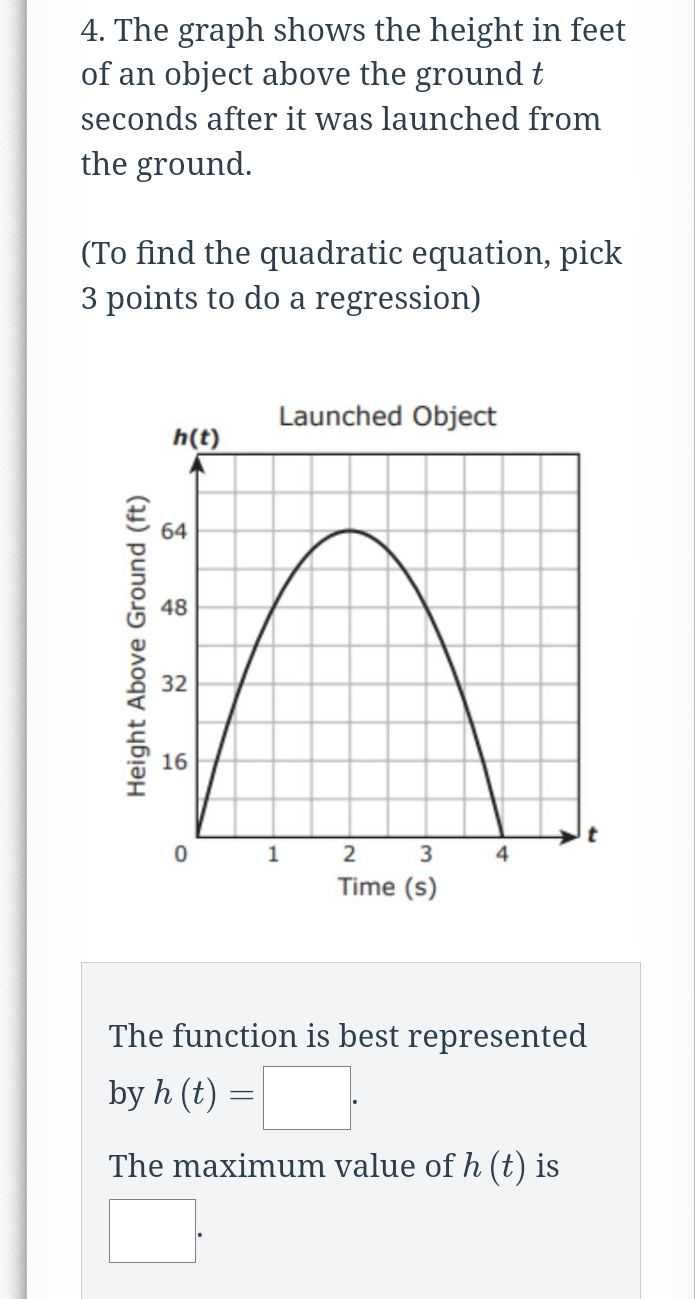### ¿Todavía tienes preguntas de matemáticas?

Pregunte a nuestros tutores expertos
Algebra
Pregunta4. The graph shows the height in feet of an object above the ground $$t$$ seconds after it was launched from the ground. (To find the quadratic equation, pick $$3$$ points to do a regression)

The function is best represented by $$h ( t ) =$$

The maximum value of $$h ( t )$$ is

$$- 16t^2+ 64t$$